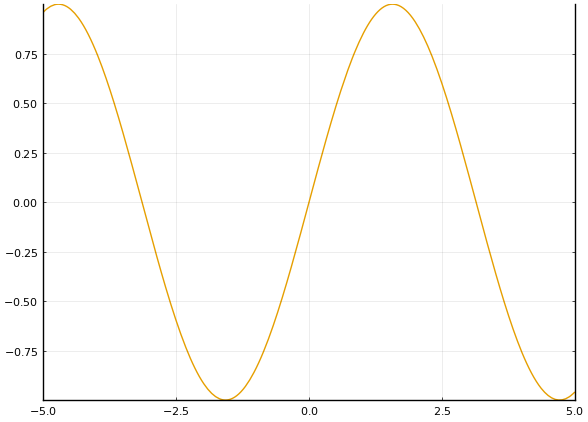# Remove top or bottom axis in :box plot

Hi there,

I’ve been searching for a way of doing this, but cannot find anything…

I’ve been trying to make a box plot but with a missing axis. Essentially, I would like to get the results given by

``````plot(framestyle = :box)
``````

but with the top axis missing (or the bottom one missing). I can remove both the top and bottom x-axis at once, by doing something like

``````plot(framestyle = :box, xaxis = false)
``````

but I would like to only remove either the top or the bottom.

Is there a way to do this?

Thanks!

Try something like:

``````using Plots
p = plot(sin, framestyle=:axis, widen=false)
vline!([xlims(p)], lc=:black, lw=2)
``````

Hmm, yeah that could work, but I wonder if there is not a buit-in way of doing that.

Especially if I’d like to have tick marks on both sides and stuff like that.

But thanks for the idea!

The `:box` frame style doesn’t seem to provide tick marks on both sides, at least on GR backend.

Oh yeah, you’re totally right, I should’ve specified.

I’m using the PyPlot backend. You get tick marks on that backend.

``````pyplot()
plot(framestyle = :box)
``````

produces

Ok, for such partial boxing using Plots.jl you could do:

``````using Plots; pyplot(dpi=100)   # or:  gr(), etc.
p = plot(sin, framestyle=:axes, widen=false)
plot!(twinx(p), grid=:on, ylims=ylims(p), yformatter=_->"")
``````Oh awesome! That’s what I was looking for.

Thanks!